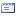Roads and Bridges - Drogi i Mosty
1, 2, 2002, 53-83

### Vibration of elastic beam forced by linear one-mass moving oscillator

Wacław SzcześniakWarsaw University of Technology, Faculty of Civil Engineering
Magdalena AtamanWarsaw University of Technology, Faculty of Civil Engineering
Artur ZbiciakWarsaw University of Technology, Faculty of Civil Engineering
Published: 2014-12-07

#### Abstract

The work presented herein can be used by engineers and designers for evaluation the difference between static and dynamic deflection of bridge constructions. The paper deals with the problem of one-mass oscillator moving on the Bernoulli-Euler beam and consists of two parts: part l - problem formulation, part II - numerical examples. The problem formulation is presented and the system of differential equations is obtained analytically in the part l. It contains five paragraphs: introduction, equations of motion of one-mass oscillator and beam, free vibrations of beam and oscillator, problem analysis in case of greater number of terms of the series, conclusions, literature. In the first paragraph the aim of the paper and the literature review are presented. Equations of motion of beam and oscillator are derived in the paragraph 2. These are differential, quadratic equations with variable coefficients. Analytical solutions of such equations are unknown. Dynamic pressure of oscillator to the beam is presented in two alternative ways (3). The first approximation of equation of beam motion solution is one term ofthe series (5). The solution of differential equations system is provided by means of Bubnov-Galerkin method. Following dimensionless co-ordinates and quantities are introduced. Finally this leads to the system of dimensionless equations of motion (20). Free vibrations of oscillator and beam are presented in the paragraph 3. The next paragraph is devoted to the beam dynamic analysis taking into account i-terms of the series (29). When the series formula is limited to three terms, system of four differential equations is obtained (30). This system of equations of motion includes four dimensionless unknowns. These are conjugated, quadratic differential equations with variable coefficients. The two last paragraphs contain conclusions and literature. The programme for oscillator and beam vibrations simulation as well as influence of various parameters on the character of vibrations are presented in the 2nd part of the paper. The oscillator is a simplified model of a truck. Both beam and oscillator are modelled as a Kelvin-Voigt's bodies. Because it is impossible to solve the problem by analytical method, it was done using the ode45 function implemented in MATLAB package. Additionally the programme for simulation of oscillator and beam vibrations has been created. The influence of various dimensionless parameters on the vibrations character is presented. Figures 4-16 show deflection of the midpoint of the span. It was investigated that the point of the maximum deflection value has a tendency to move toward the departure-end-time of the beam (see Fig. 14-16). In the Fig. 17 the curve of the dynamical pressure is visualised. It can be noted that in the case of non-zero damping parameter value in oscillator the pressure curve is discontinuous. Figure 18 shows the history curve of each beam's points deflection under moving oscillator.

### Vibration of elastic beam forced by linear one-mass moving oscillator

Szcześniak, Wacław; Ataman, Magdalena; Zbiciak, Artur. Vibration of elastic beam forced by linear one-mass moving oscillator. Roads and Bridges - Drogi i Mosty, [S.l.], v. 1, n. 2, p. 53-83, dec. 2014. ISSN 2449-769X. Available at: <>. Date accessed: 04 Oct. 2023.